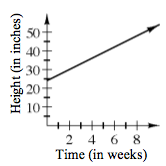### Home > INT3 > Chapter Ch1 > Lesson 1.1.4 > Problem1-58

1-58.

Gregory planted a lemon tree in his back yard. When he planted the tree, it was two feet tall. He notices that it has been growing three inches every week.

1. Create multiple representations to represent the relationship between the days that have passed and the height of the tree.

Remember, there are $12$ inches in a foot.

Start with a table. How tall is the tree on day $0$, the day it was planted? Use what you know about how it grows to generate the rest of the table.

$\left. \begin{array} { c | c } { x } & { y } \\ \hline 0 & { 24 } \\ { 1 } & { 27 } \\ { 2 } & { 30 } \\ { 3 } & { 33 } \\ { 4 } & { 36 } \\ { 5 } & { 39 } \end{array} \right.$$y = 3x + 24$

2. If the tree continues growing at this rate, when will it be six feet tall? How can you see this growth in each of the representations?

How many inches are there in $6$ feet?

Where do you see that on the table? On the graph? Describe it.

Substitute $72$ for $y$ in the equation and solve for $x$.

$16$ weeks

3. State the possible inputs and outputs of the graph.

Possible inputs: all number greater than and including $0$
Possible outputs: all numbers greater than and including $24$

Use the blank graph in the eTool below to help solve the problem.
Click the link at right for the full version of the eTool: Int3 1-58 HW eTool